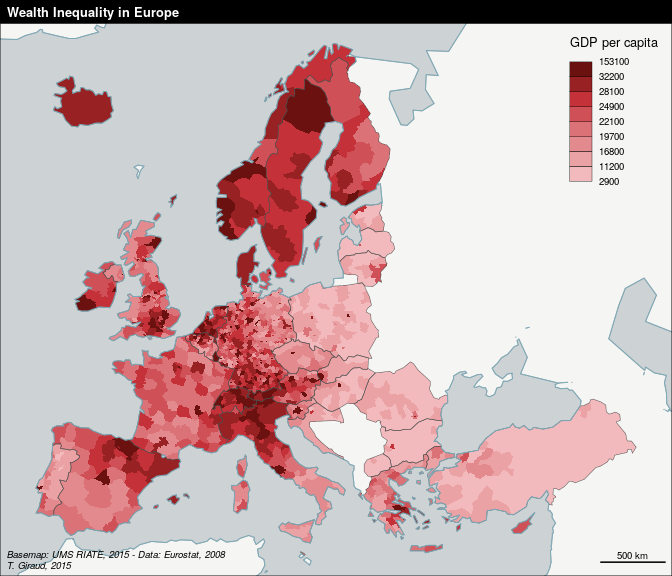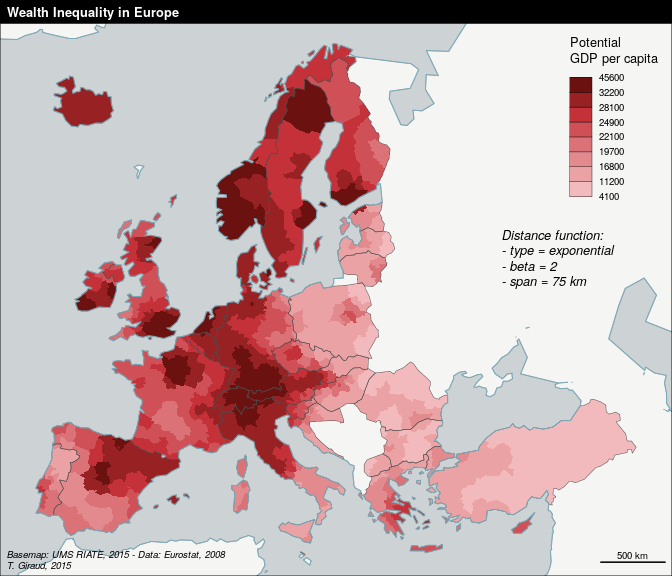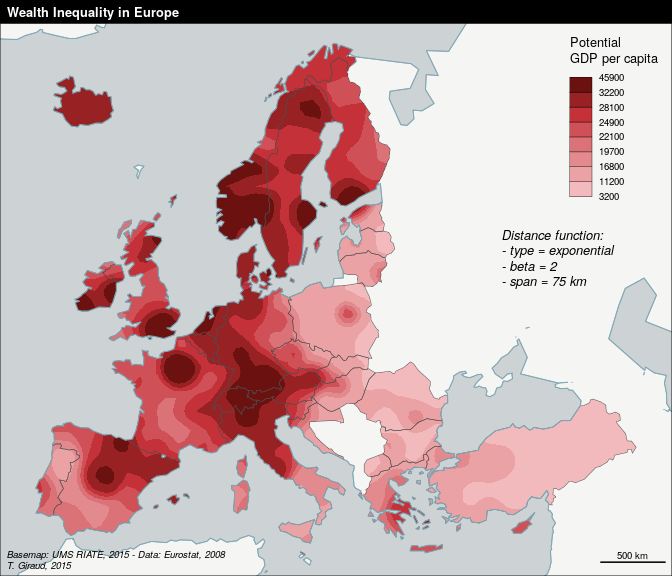# Stewart Potentials: a Use Case

#### 2019-05-10

The Stewart potentials of population is a spatial interaction modeling approach which aims to compute indicators based on stock values weighted by distance. These indicators have two main interests:

1. they produce understandable maps by smoothing complex spatial patterns;
2. they enrich the stock variables with contextual spatial information.

At the European scale, this functional semantic simplification may help to show a smoothed context-aware picture of the localized socio-economic activities.

In this vignette, we show a use case of these “potentials” on the regional GDP per capita at the European scale with three maps:

• a regional map of the GDP per capita;
• a regional map of the potential GDP per capita;
• a smoothed map of the GDP per capita.

Note that this example is based on data and mapping functions proposed in the cartography package.

## Regional Map of the GDP per Capita

library(cartography)
library(sp)
library(sf)
library(SpatialPosition)
data(nuts2006)

# Compute the GDP per capita variable
nuts3.df$gdpcap <- nuts3.df$gdppps2008 * 1000000 / nuts3.df$pop2008 # Discretize the variable bv <- quantile(nuts3.df$gdpcap, seq(from = 0, to = 1, length.out = 9))

# Draw the map
opar <- par(mar = c(0,0,1.2,0))

# Set a color palette
pal <- carto.pal(pal1 = "wine.pal", n1 = 8)

# Draw the basemap
plot(nuts0.spdf, add = F, border = NA, bg = "#cdd2d4")
plot(world.spdf, col = "#f5f5f3ff", border = "#a9b3b4ff", add = TRUE)

# Map the regional GDP per capita
choroLayer(spdf = nuts3.spdf, df = nuts3.df,
var = "gdpcap",
legend.pos = "topright",
breaks = bv, col = pal,
border = NA,
legend.title.txt = "GDP per capita",
legend.values.rnd = -2,
add = TRUE)
plot(nuts0.spdf, add = TRUE, lwd = 0.5, border = "grey30")
plot(world.spdf, col = NA, border = "#7DA9B8", add = TRUE)

# Set a layout
layoutLayer(title = "Wealth Inequality in Europe",
sources = "Basemap: UMS RIATE, 2015 - Data: Eurostat, 2008",
author = "T. Giraud, 2015")par(opar)

## Regional Map of the Potential GDP per Capita

We compute the potentials of GDP for each spatial unit. The computed value takes into account the spatial distribution of the stock variable and return a sum weighted by distance, according a specific spatial interaction and fully customizable function.

# Create a distance matrix between units
mat <- CreateDistMatrix(knownpts = nuts3.spdf,
unknownpts = nuts3.spdf)

# Merge the data frame and the SpatialPolygonsDataFrame
nuts3.spdf@data <- nuts3.df[match(nuts3.spdf$id, nuts3.df$id),]

# Compute the potentials of population per units
# function = exponential, beta = 2, span = 75 km
poppot <- stewart(knownpts = nuts3.spdf,
unknownpts = nuts3.spdf,
matdist = mat,
varname = "pop2008",
typefct = "exponential",
beta = 2,
span = 75000,
returnclass = "sf")

# Compute the potentials of GDP per units
# function = exponential, beta = 2, span = 75 km
gdppot <- stewart(knownpts = nuts3.spdf,
unknownpts = nuts3.spdf,
matdist = mat,
varname = "gdppps2008",
typefct = "exponential",
beta = 2,
span = 75000,
returnclass = "sf")

# Create a data frame of potential GDP per capita
pot <- data.frame(id = nuts3.df$id, gdpcap = gdppot$OUTPUT * 1000000 / poppot$OUTPUT, stringsAsFactors = FALSE) # Discretize the variable bv2 <- c(min(pot$gdpcap), bv[2:8], max(pot$gdpcap)) # Draw the map par <- par(mar = c(0,0,1.2,0)) # Draw the basemap plot(nuts0.spdf, add = F, border = NA, bg = "#cdd2d4") plot(world.spdf, col = "#f5f5f3ff", border = "#a9b3b4ff", add = TRUE) # Map the regional potential of GDP per capita choroLayer(spdf = nuts3.spdf, df = pot, var = "gdpcap", legend.pos = "topright", breaks = bv2, col = pal, border = NA, legend.title.txt = "Potential\nGDP per capita", legend.values.rnd = -2, add = TRUE) plot(nuts0.spdf, add=T, lwd = 0.5, border = "grey30") plot(world.spdf, col = NA, border = "#7DA9B8", add=T) # Set a text to explicit the function parameters text(x = 6271272, y = 3743765, labels = "Distance function:\n- type = exponential\n- beta = 2\n- span = 75 km", cex = 0.8, adj = 0, font = 3) # Set a layout layoutLayer(title = "Wealth Inequality in Europe", sources = "Basemap: UMS RIATE, 2015 - Data: Eurostat, 2008", author = "T. Giraud, 2015")par(opar) This map gives a smoothed picture of the spatial patterns of wealth in Europe while keeping the original spatial units as interpretive framework. Hence, the map reader can still rely on a known territorial division to develop its analyses. ## Smoothed Map of the GDP per Capita In this case, the potential GDP per capita is computed on a regular grid. # Compute the potentials of population on a regular grid (50km span) # function = exponential, beta = 2, span = 75 km poppot <- stewart(knownpts = nuts3.spdf, varname = "pop2008", typefct = "exponential", span = 75000, beta = 2, resolution = 50000, mask = nuts0.spdf, returnclass = "sf") # Compute the potentials of GDP on a regular grid (50km span) # function = exponential, beta = 2, span = 75 km gdppot <- stewart(knownpts = nuts3.spdf, varname = "gdppps2008", typefct = "exponential", span = 75000, beta = 2, resolution = 50000, mask = nuts0.spdf, returnclass = "sf") # Create the ratio variable poppot$OUTPUT2 <- gdppot$OUTPUT * 1e6 / poppot$OUTPUT

# Create an isopleth layer
pot <- isopoly(x = poppot, var = "OUTPUT2",
breaks = bv,
mask = nuts0.spdf,
returnclass = "sf")

# Get breaks values
bv3 <- sort(c(unique(pot$min), max(pot$max)), decreasing = FALSE)

# Draw the map
par <- par(mar = c(0,0,1.2,0))

# Draw the basemap
plot(nuts0.spdf, add = F, border = NA, bg = "#cdd2d4")
plot(world.spdf, col = "#f5f5f3ff", border = "#a9b3b4ff", add = TRUE)

# Map the potential GDP per Capita
choroLayer(x = pot, var = "center",
legend.pos = "topright",
breaks = bv3, col = pal, add=T,
border = NA, lwd = 0.2,
legend.title.txt = "Potential\nGDP per capita",
legend.values.rnd = -2)
plot(nuts0.spdf, add=T, lwd = 0.5, border = "grey30")
plot(world.spdf, col = NA, border = "#7DA9B8", add=T)

# Set a text to explicit the function parameters
text(x = 6271272, y = 3743765,
labels = "Distance function:\n- type = exponential\n- beta = 2\n- span = 75 km",
cex = 0.8, adj = 0, font = 3)

# Set a layout
layoutLayer(title = "Wealth Inequality in Europe",
sources = "Basemap: UMS RIATE, 2015 - Data: Eurostat, 2008",
author = "T. Giraud, 2015")par(opar)

Unlike the previous maps, this one doesn’t keep the initial territorial division to give a smoothed picture of the spatial patterns of wealth in Europe. The result is easy to read and can be considered as a bypassing of the Modifiable Areal Unit Problem (MAUP).